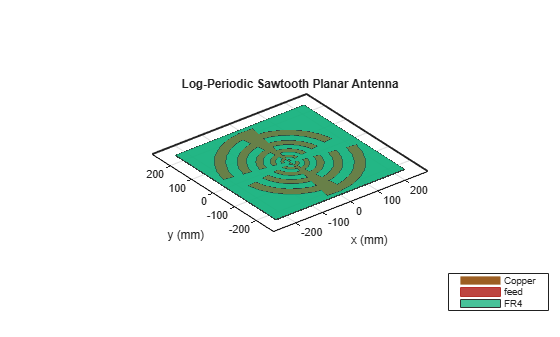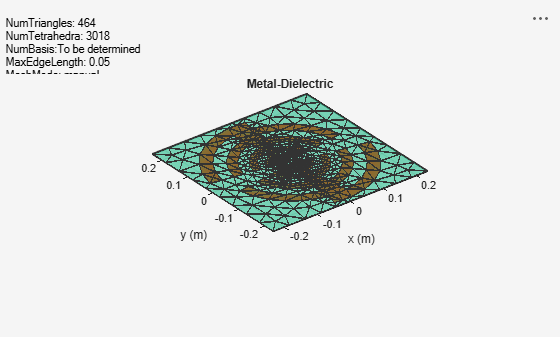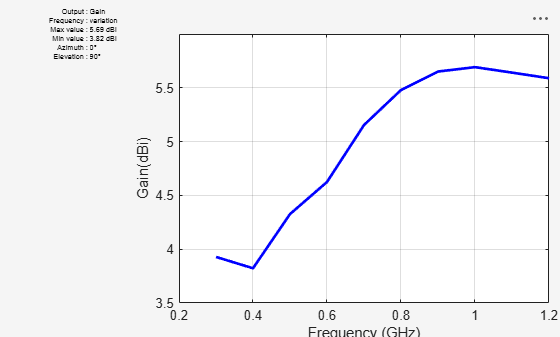# Design Log-Periodic Sawtooth Planar Antenna for UHF Ultra-Wideband Applications

This example shows how to create, model, and analyze two-arm log-periodic sawtooth planar microstrip antenna . It is a bowtie-like antenna that consists of two arms located on the square-shaped FR4 dielectric substrate. This example designs a log-periodic sawtooth planar antenna with stable impedance over a wide bandwidth from 300 MHz to 1200 GHz. This antenna is widely used in the ultra high frequency (UHF) range and for ultra-wideband (UWB) systems such as ground penetrating radar systems (GPRs). The non-evasive and nondestructive technique in GPRs uses electromagnetic radiation in the microwave band. GPRs use high-frequency radio waves, usually in the 10 MHz to 2.6 GHz range.### Define Parameters

The dimensions and the variables used in this example are taken from the paper . The variables `r` and `d` specify the radius and width of the arms of the log-periodic antenna. The `Angle` variable is used as an `ArcAngle` for creating the arms of the antenna. The `r`, `d`, and `Angle` variables are used to design the arms on either side of the center arc. The `NumPoints` variable is used to specify the number of discretization points.

```Angle = 18; r = [199 166 136 109 85 64 46 31 19 5]*1e-3; d = [33 30 27 24 21 18 15 12 9 10]*1e-3; NumPoints = 8;```

### Create Log-Periodic Shape

Use the `generatePointsforCurve `function to create the vertices for the arms of the antenna. This function takes the angle, radius, width, and number of discretization points as the inputs and generates the boundary vertices for the curves. These vertices are used to form an arm using the `antenna.polygon `function. All the arms on either side of the center arc can be generated by using `r` and `d` variables. All the polygons formed are combined to form the upper half of the log-periodic antenna.

```NumShapes = numel(1,r); p1 = generatePointsforCurve([90-Angle/2,90+Angle/2],(205e-3)/2,205e-3,NumPoints/2); shp = antenna.Polygon('Vertices',p1'); for i=1:NumShapes if rem(i,2)==1 tempPoints = generatePointsforCurve([90-Angle/2,180-Angle/2],r(i),d(i),NumPoints); tempShape = antenna.Polygon('Vertices',tempPoints'); shp = shp+tempShape; else tempPoints = generatePointsforCurve([Angle/2,90+Angle/2],r(i),d(i),NumPoints); tempShape = antenna.Polygon('Vertices',tempPoints'); shp = shp+tempShape; end end```

Shift the shape along the y-axis to create a gap for the feed using the `translate` function. The total gap given in  is 10 mm, so shift the upper half of the shape by 5 mm.

`shp = translate(shp,[0 5e-3 0]);`

Copy the shape into a variable `shp1` and use it to create a symmetrical shape. Use the `rotateZ` function to rotate the shape replica by 180 degrees.

```shp1 = copy(shp); shp1 = rotateZ(shp1,180);```

Create a rectangle for the feed 0.5 mm in length and 15 mm in width using the `antenna.Rectangle` shape object.

`feed = antenna.Rectangle('Length',0.5e-3,'Width',15e-3);`

Unify shapes `shp` and `shp1` with the `feed` to form sawtooth-shaped antenna structure.

```shape = shp+shp1+feed; figure; show(shape)```### Create Log-Periodic Antenna Using PCB Stack

Create a `pcbStack` with two layers. The top metal layer is the log-periodic shape antenna and the bottom layer is the square-shaped dielectric substrate FR4 of length `470` mm. The substrate has a dielectric constant of `4.6`, an attenuation coefficient of `0.02`, and the substrate thickness of `1.6` mm. The top conductor is set to copper with a thickness of `35` um.

```ant = pcbStack; d = dielectric('FR4'); d.Thickness = 1.6e-3; BoardDim = antenna.Rectangle('Length',470e-3,'Width',470e-3); ant.Name = 'LogPeriodic'; ant.Layers = {shape,d}; ant.BoardShape = BoardDim; ant.FeedLocations = [0 0 1]; ant.BoardThickness = 1.6e-3; ant.FeedDiameter = 0.25e-3; ant.FeedViaModel = 'strip'; ant.Conductor = metal('copper'); ant.Conductor.Thickness = 35e-6;```

Use the `show` function to visualize the antenna.

```figure; show(ant)```### Meshing Antenna

Mesh the structure by specifying the maximum edge length. Below is the mesh used to model the antenna. The triangles are used to discretize the metal regions of the patch, and tetrahedra are used to discretize the volume of the dielectric substrate in the antenna. The triangles and tetrahedra are indicated by the colors yellow and green, respectively. The total number of unknowns is the sum of the unknowns for the metal plus the unknowns used for the dielectric. As a result, the time to calculate the solution increases significantly as compared to pure metal antennas. Set the maximum edge length to 0.05. This value can be decreased to generate a denser mesh.

```figure; mesh(ant,'MaxEdgeLength',0.05)```### Analyze Antenna

Plot the impedance of the antenna using the `impedance` function over a frequency range of 100-1250 MHz.

```figure; impedance(ant,100e6:10e6:1250e6);```The impedance plot shows that real part of the impedance is approximately 220 ohms over the specified range. And the imaginary part of the impedance is almost 0. The results in  are obtained by using a balun to transform the impedance to 50 ohms. Set the reference impedance as 220 ohms to obtain results similar to . Plot S-parameters over a frequency 300-1250MHz with the reference impedance of 220 ohms using the `sparameters` function. The antenna has an S11 of value less than -10 dB over a frequency range of 300-1200 MHz.

```spar = sparameters(ant,100e6:10e6:1250e6,220); figure; rfplot(spar)```Use the `pattern` function to plot the radiation pattern of the antenna at a frequency of 600 MHz.

```figure; pattern(ant,600e6)```Plot the radiation pattern of the antenna in the rectangular cartesian coordinate system over a frequency range of 300-1200 MHz

```figure; pattern(ant,300e6:100e6:1200e6,0,90,'CoordinateSystem','rectangular')```### Conclusion

Modelling and analysis of a log-periodic antenna using the Antenna Toolbox is completed and its performance matches the results given in . The return loss (S11) of the antenna indicates a wideband performance over a frequency range of 300-1200 MHz. The antenna has an approximate gain of 4.94 dBi at 600 MHz and the gain increases with the increase in frequency.

### Reference

. Phu B. H., Quang P. M., Phuoc D. T. and Duy L. N., "A log-periodic saw-toothed planar antenna for UHF ultra-wideband applications," 2013 International Conference on Advanced Technologies for Communications, 2013, pp. 689-692.